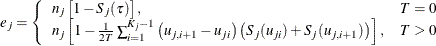#### Analyses in the TWOSAMPLESURVIVAL Statement

##### Rank Tests for Two Survival Curves (TEST=LOGRANK, TEST=GEHAN, TEST=TARONEWARE)

The method is from Lakatos (1988) and Cantor (1997, pp. 83–92).

Define the following notation: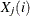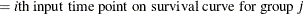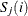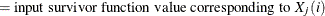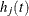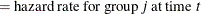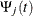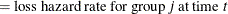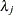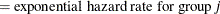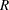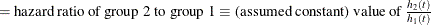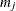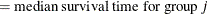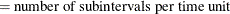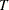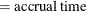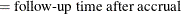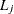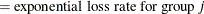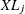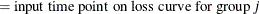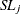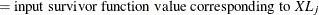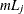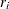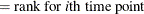Each survival curve can be specified in one of several ways.

• For exponential curves:

• a single point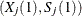on the curve

• median survival time

• hazard rate

• hazard ratio (for curve 2, with respect to curve 1)

• For piecewise linear curves with proportional hazards:

• a set of points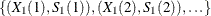(for curve 1)

• hazard ratio (for curve 2, with respect to curve 1)

• For arbitrary piecewise linear curves:

• a set of points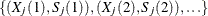A total of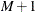evenly spaced time points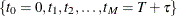are used in calculations, where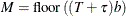The hazard function is calculated for each survival curve at each time point. For an exponential curve, the (constant) hazard is given by one of the following, depending on the input parameterization: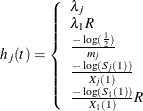For a piecewise linear curve, define the following additional notation: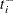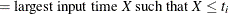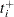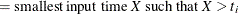The hazard is computed by using linear interpolation as follows: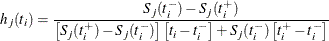With proportional hazards, the hazard rate of group 2’s curve in terms of the hazard rate of group 1’s curve is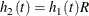Hazard function values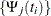for the loss curves are computed in an analogous way from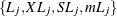.

The expected number at risk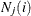at time i in group j is calculated for each group and time points 0 through M – 1, as follows: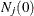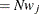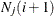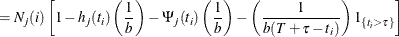Define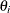as the ratio of hazards and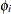as the ratio of expected numbers at risk for time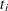: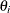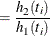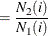The expected number of deaths in each subinterval is calculated as follows: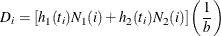The rank values are calculated as follows according to which test statistic is used: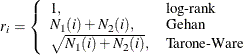The distribution of the test statistic is approximated by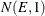where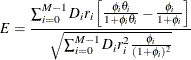Note that
can be factored out of the mean E, and so it can be expressed equivalently as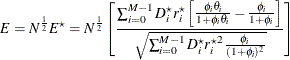where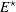is free of N and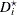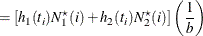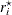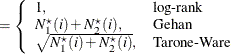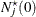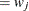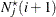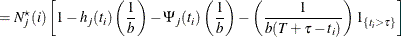The approximate power is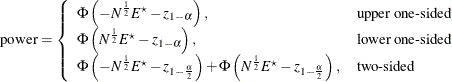Note that the upper and lower one-sided cases are expressed differently than in other analyses. This is because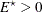corresponds to a higher survival curve in group 1 and thus, by the convention used in PROC power for two-group analyses, the lower side.

For the one-sided cases, a closed-form inversion of the power equation yield an approximate total sample size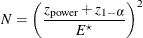For the two-sided case, the solution for N is obtained by numerically inverting the power equation.

Accrual rates are converted to and from sample sizes according to the equation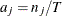, where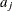is the accrual rate for group j.

Expected numbers of events—that is, deaths, whether observed or censored—are converted to and from sample sizes according to the equation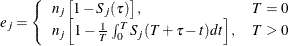where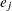is the expected number of events in group j. For an exponential curve, the equation simplifies to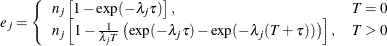For a piecewise linear curve, first define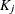as the number of time points in the following collection:,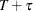, and input time points for group j strictly betweenand. Denote the ordered set of these points as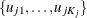. The survival function values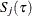and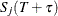are calculated by linear interpolation between adjacent input time points if they do not coincide with any input time points. Then the equation for a piecewise linear curve simplifies to